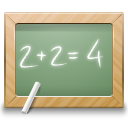Subsections

align_tensor.matrix_anglesSynopsis

Calculate the angles between all alignment tensors.

Defaults

align_tensor.matrix_angles(basis_set=`matrix', tensors=None, angle_units=`deg', precision=1)

Keyword arguments

basis_set: The basis set to operate with.

tensors: A list of the tensors to apply the calculation to. If None, all tensors are used.

angle_units: The units for the angle parameters, either `deg' or `rad'.

precision: The precision of the printed out angles. The number corresponds to the number of figures to print after the decimal point.

Description

This will calculate the inter-matrix angles between all loaded alignment tensors for the current data pipe. For the vector basis sets, the matrices are first mapped to vector form and then then the inter-vector angles rather than inter-matrix angles are calculated. The angles are dependent upon the basis set - linear maps produce identical results whereas non-linear maps result in different angles. The basis set can be one of:

`matrix' -
The standard inter-matrix angles. This default option is a linear map, hence angles are preserved. The angle is calculated via the arccos of the Euclidean inner product of the alignment matrices in rank-2, 3D form divided by the Frobenius norm ||A||_F of the matrices.
`irreducible 5D' -
The inter-tensor vector angles for the irreducible spherical tensor 5D basis set {A-2, A-1, A0, A1, A2}. This is a linear map, hence angles are preserved. These are the spherical harmonic decomposition coefficients.
`unitary 9D' -
The inter-tensor vector angles for the unitary 9D basis set {Sxx, Sxy, Sxz, Syx, Syy, Syz, Szx, Szy, Szz}. This is a linear map, hence angles are preserved.
`unitary 5D' -
The inter-tensor vector angles for the unitary 5D basis set {Sxx, Syy, Sxy, Sxz, Syz}. This is a non-linear map, hence angles are not preserved.
`geometric 5D' -
The inter-tensor vector angles for the geometric 5D basis set {Szz, Sxxyy, Sxy, Sxz, Syz}. This is a non-linear map, hence angles are not preserved. This is also the Pales standard notation.

The full matrix angle via the Euclidean inner product is defined as

/   <A1 , A2>   \
theta = arccos | ------------- | ,
\ ||A1||.||A2|| /

where <a,b> is the Euclidean inner product and ||a|| is the Frobenius norm of the matrix. For the irreducible spherical tensor 5D basis set, the Am components are defined as

/ 4pi \ 1/2
A0 = | --- |     Szz ,
\  5  /

/ 8pi \ 1/2
A+/-1 = +/- | --- |     (Sxz +/- iSyz) ,
\ 15  /

/ 2pi \ 1/2
A+/-2 = | --- |     (Sxx - Syy +/- 2iSxy) ,
\ 15  /

and, for this complex notation, the angle is

/ Re(<A1|A2>) \
theta = arccos | ----------- | ,
\  |A1|.|A2|  /

where the inner product is defined as

___
\      1    2*
<A1|A2> =   >   Am . Am   ,
/__
m=-2,2

and where Am* = (-1)^m A-m, and the norm is defined as |A1| = Re(sqrt(<A1|A1>)). For all other basis sets whereby the map is real matrix -> real vector, the inter-tensor angle is defined as

/  <A1|A2>  \
theta = arccos | --------- | ,
\ |A1|.|A2| /

where the inner product <A1|A2> is simply the vector dot product and |A1| is the vector length.

The relax user manual (PDF), created 2020-08-26.